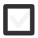# Solve a problem by drawing a FIGURE. Group data, find patterns & solve the parts.108 views
In 7-9
edited

## 1 lesson

+1 vote
by Guru (6.1k)
edited

Problem solving strategy

When one comes across a prblem that seems like it's too hard to solve, many give up. However, when equipped with the right strategies, there's no problem in this world that is impossible. As the saying goes, an elephant is eaten one little piece at a time and the same goes for probolem solving. Have a look at the steps below that are meant for solving typical school math written problems.

2. What is the QUESTION? Highlight it.
3. What is known? Circle all pieces of information there are.
4. Make a drawing of the problem.
5. How can you solve the problem?
6. Write an expression
7. Calculate